# GMAT Math : Calculating the perimeter of a square

## Example Questions

### Example Question #1 : Calculating The Perimeter Of A Square

A square plot of land has area 256 square yards. Give its perimeter in inches.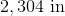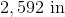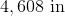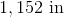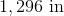Explanation:

The sidelength of a square is the square root of its area - in this case,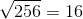yards. Its perimeter is therefore four times that, or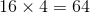yards. Multiply by 36 to convert to inches: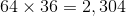inches.

### Example Question #2 : Calculating The Perimeter Of A Square

Five squares have sidelengths 3, 4, 5, 6, and 7 meters. What is the mean of their perimeters?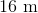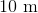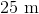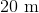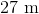Explanation:

Multiply each sidelength by four to get the perimeters - they will be 12, 16, 20, 24, and 28 meters, respectively. The mean will be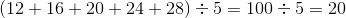meters

### Example Question #3 : Calculating The Perimeter Of A Square

Given Square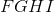, answer the following questions.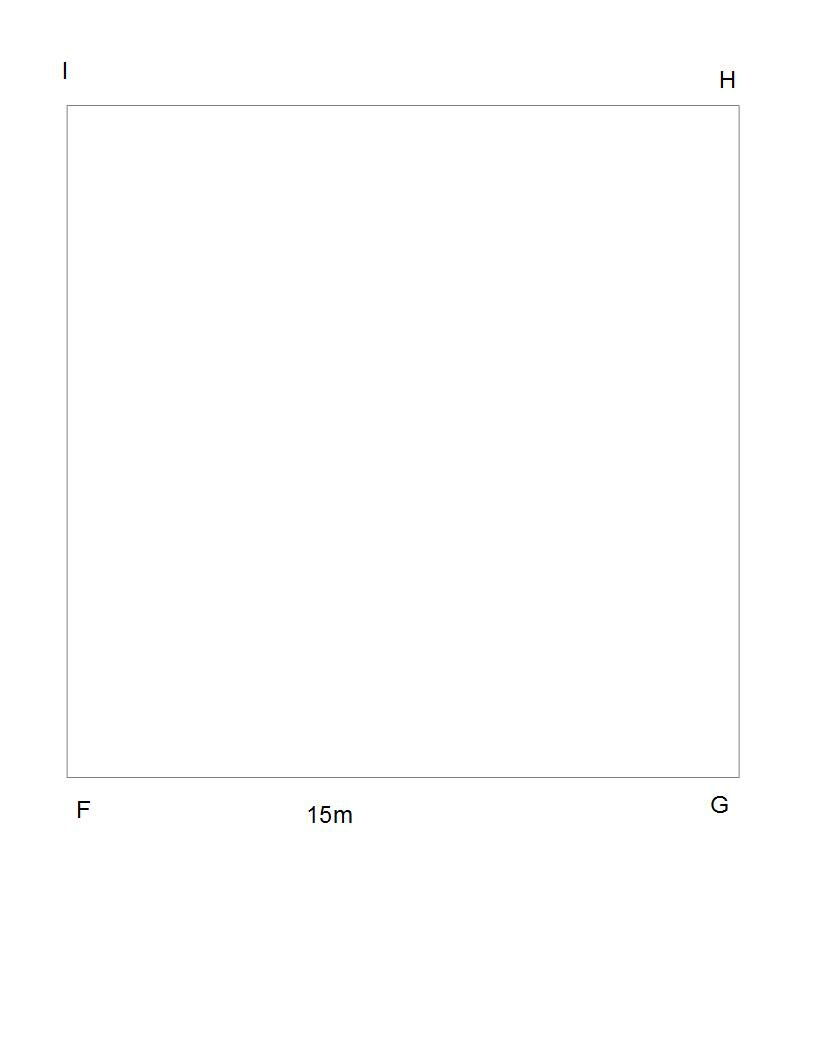Squarerepresents a small field for a farmer's sheep. How many meters of fence will the farmer require to completely enclose the field?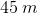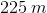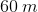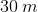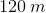Explanation:

This question is a thinly veiled perimeter of a square question. To find the total amount of fencing needed, use the following formula: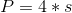Whereis the perimeter of a square, andis the length of one side.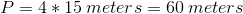### Example Question #4 : Calculating The Perimeter Of A Square

A given square has a side length of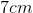. What is its perimeter?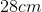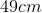Not enough information provided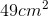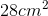Explanation:

In order to find the perimeterof a given square with side length, we use the equation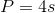. Given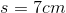, we can therefore conclude that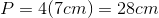### Example Question #5 : Calculating The Perimeter Of A Square

A given square has an area of. What is its perimeter?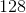Not enough information providedExplanation:

We are told that the areaof the square is. We know the area of a square is defined as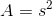, whereis the length of the side of the square. We can therefore deduce that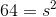and that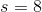In order to find the perimeterof a given square with side length, we use the equation. Given, we can therefore conclude that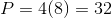.

### Example Question #6 : Calculating The Perimeter Of A Square

A given square has a side of length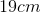. What is the perimeter of the square?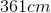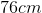Not enough information provided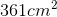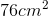Explanation:

In order to find the perimeterof a given square with side length, we use the equation. Given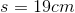, we can therefore conclude that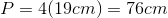### Example Question #7 : Calculating The Perimeter Of A Square

The area of a square is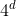. Give its perimeter.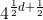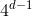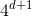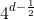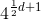Explanation:

The length of one side of a square is the square root of the area - or, equivalently, the area raised to the power of. Here, this is.

Multiply this by 4 to get the perimeter: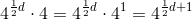### Example Question #8 : Calculating The Perimeter Of A Square

A square courtyard will have paths running diagonally across it (the paths will form an x). If the length of one of the paths will be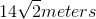long, what will the perimeter of the courtyard be?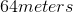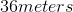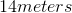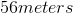Explanation:

A square courtyard will have paths running diagonally across it (the paths will form an x). If the length of one of the paths will belong, what will the perimeter of the courtyard be?

To find the perimeter of a square, we need to find the side lengths. We can find the side lengths from the diagonal by recalling that the diagonal of a square creates two 45/45/90 triangles.

A 45/45/90 triangle has the following side-length ratios: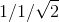This means that if our hypotenuse islong, our other two sides will be 14 meters long

So our side-length is 14 meters, find the perimeter via the following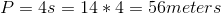### Example Question #9 : Calculating The Perimeter Of A Square

A square is created from joining two equilateral triangles of side length =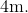What is the perimeter of the square?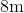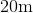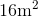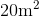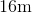Explanation:

Draw yourself a picture for this problem.  If you create a square out of two triangles, they meet at the diagonal of the square.  Since, we know the triangles are equilateral, then all of the side lengths of the triangle and square are equal.  Therefore, we just take the side length and add that four times.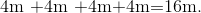Tired of practice problems?

Try live online GMAT prep today.